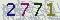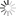﻿ Necessary and  sufficient  conditions to  solve  parabolic Anderson  model with rough noise-山东大学新闻网### Necessary and  sufficient  conditions to  solve  parabolic Anderson  model with rough noise

Necessary and sufficient conditions to solve parabolic Anderson model with rough noise

2022年7月6日9:30-11:30

We obtain necessary and sufficient conditions for the existence of $n$-th chaos of the solution to the parabolic Anderson model $\frac{\partial}{\partial t}u(t,x)=\frac{1}{2}\Delta u(t,x)+u(t,x)\dot{W}(t,x)$, where $\dot{W}(t,x)$ is a fractional Brownian field with temporal Hurst parameter $H_0\ge 1/2$ and spatial parameters $H$ $=(H_1, \cdots, H_d)$ $\in (0, 1)^d$.

When $d=1$, we extend the condition on the parameters under which the chaos expansion of the solution is convergent in the mean square sense, which is both sufficient and necessary under some circumstances.

Xiong Wang otained his PhD degree at University of Alberta in June 2022. His current research focuses on stochastic partial differential equations (SPDEs) and stochastic differential equations (SDEs).

【作者：杨媛    来自：数学与交叉科学研究中心    编辑：新闻网工作室    责任编辑：周长瑞 蒋晓涵 　】

 验证码看不清楚,换张图片 共0条评论    共1页   当前第1页 拖动光标可翻页查看更多评论

### 最新发布### 新闻排行• 山大日记
• 山大人物
• 视点微信
• 互动话题
• 视点图志
• 精彩视频